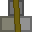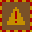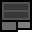# Account 984526

## BalanceMetal: 20s5 = 325Explosives: 1s0 = 16RC Turrets: 2

## Transfer

 PIN Receiver ReferenceMetal 16 × +Explosives 16 × +RC Turrets

## Create new account token

PIN:

New accounts cost 2 stacks (32 units) metal. You must give the token directly to the other player. Do not create the account yourself and give them the details. If you are found to do this, your account will be closed and all your items taken. You are responsible for ensuring the player you provide a new account token to knows how to use dredbank properly. If many players are found who don't know how to use dredbank properly, and they all got their accounts from one player, there may be a punishment against the player who provided their accounts.

## Transactions

Type Counterparty Account Reference NumberMetalExplosivesRC Turrets
Sent 220022 0 1s0 = 16 0s0 = 0 0
Sent 220022 0 2s0 = 32 0s0 = 0 0
Sent 220022 0 30s0 = 480 0s0 = 0 0
Sent 220022 0 10s0 = 160 0s0 = 0 0
Sent 333000 0 1s14 = 30 0s0 = 0 0
Deposit 0 4s0 = 64 0s0 = 0 0
Deposit 0 20s0 = 320 0s0 = 0 2
Deposit 0 26s0 = 416 0s0 = 0 0
Sent 927415 0 0s0 = 0 2s0 = 32 0
Deposit 0 15s3 = 243 3s0 = 48 0
Sent 789542 0 6s0 = 96 1s0 = 16 0
Deposit 0 3s0 = 48 1s0 = 16 0
Sent 653090 0 6s0 = 96 0s0 = 0 0
Sent 653090 0 0s12 = 12 0s0 = 0 0
Deposit 0 6s0 = 96 0s0 = 0 0
Sent 653090 0 0s1 = 1 0s0 = 0 0
Deposit 0 3s0 = 48 0s0 = 0 0
Sent 653090 0 0s1 = 1 0s0 = 0 0
Sent 653090 0 0s1 = 1 0s0 = 0 0
Sent 653090 0 0s1 = 1 0s0 = 0 0
Deposit 0 1s0 = 16 0s0 = 0 0